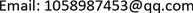CO2埋存，低渗透油藏，井筒泄漏，风险评价，模型, CO2 Storage Low Permeability Reservoir Wellbore Leakage Risk Evaluation Model

1西南石油大学油气藏地质及开发工程国家重点实验室，四川 成都

2中国石油长庆油田勘探开发研究院，陕西 西安1. 引言

2. 井筒泄漏规模与风险评价

3. CO<sub>2</sub>的泄漏模型

1) CO2的泄漏速率

Q G = Y C d Q A p M K R T G ( 2 K + 1 ) K + 1 K − 1 (1)

Y = ( P 0 P ) 1 K × [ 1 − ( P 0 P ) K − 1 K ] 1 2 × [ 2 K − 1 × ( K + 1 2 ) K + 1 K − 1 ] 1 2 (2)

2) CO2的泄漏浓度的分布

C = Q G 2 π μ σ y σ z exp ( − y r 2 2 σ y 2 ) { exp [ − ( z s + Δ h − z r ) 2 2 σ z 2 ] + exp [ − ( z s + Δ h + z r ) 2 2 σ z 2 ] } (3)

σ j , k 2 = σ j , k 2 ( t k ) − σ j , k 2 ( t k − 1 ) (4)

σ = a + b x g + d x (5)

Diffusion coefficient in horizontal directio

A0.0048280.73000.9311−72.03001.0740
B0.0010245.13680.9521−91.04071.0500
C0.0180266.52120.9776−163.41541.0200
D−0.02202172.36570.9972−2104.23531.0000
E0.0030243.92710.9897−192.99291.0100
F−0.01501905.17550.9984−1871.27041.0000

Diffusion coefficient in vertical directio

A0.2116 433.5448255.0555 463.66112.9324 2.1029128.3861 −443.90890.9750 0.04000.01~0.30 0.30~3.10
B0.0050 368.864729.4599 112.01092.9124 1.090989.0947 −373.34320.9270 0.01000.01~0.40 0.40~32.00
C0.002065.94660.9155−4.81610.92600.01~100.00
D0.1928 −5.4895385.5170 43.62520.7029 0.6430−352.3391 −7.29370.6900 0.85500.01~0.35 0.35~100.00
E0.8166 −8.7993803.2275 420.05590.5165 0.6342−782.5406 −389.83600.5100 0.64500.01~0.80 0.80~100.00
F0.6833 −5.248915.1577 350.19950.7375 0.6116−1.5122 −33.28680.1300 0.62000.01~0.50 0.50~100.00

*A级x > 3.10 km，扩散系数恒等于5.00 km；B级x > 32.00 km，扩散系数恒等于5.00 km。

3) 烟羽抬升高度

Δ H = Q n 1 2 ( d T a d Z + 0.0098 ) − 1 3 U − 1 3 (6)

Q h = 0.35 p a Q v Δ T T S = 0.35 p a Q v T S − T a T S (7)

4. 实例井筒泄漏分析

5. CO<sub>2</sub>泄漏风险评估

1) 影响范围预测

Environmental risk values of CO2 leakage at different wind speed

1.03.0

2) 泄漏风险评价

r = { r 0                                     0 < x 0 ≤ l 0 r 0 l − l 0 ( l − x 0 )         l 0 < x 0 ≤ l 0                                           x 0 > l (7)

r 0 = R 1 R 2 R 3 R 4 (8)

Risk values for different areas near leakage source

0~1001.18 × 10−5
3006.38 × 10−6
5002.15 × 10−6
20000

3) 风险分级

The risk classificatio

10−4危险性中等应采取相应措施

10−6与地震或天灾发生的概率相当不能引起人们的注意

6. 结论

H3区域CCUS实施过程中井筒泄漏风险评价表明：风速1 m/s的泄漏源点及距泄漏源点100 m处的环境风险值数量级为10−5，与中等危险等级相对应，CO2泄漏引起的事故危险性在井场附近，建议在距离井场中心50米范围内的四个方向安装四个用于监测空气中CO2浓度的实时在线监测装置，实时在线监测CO2浓度。